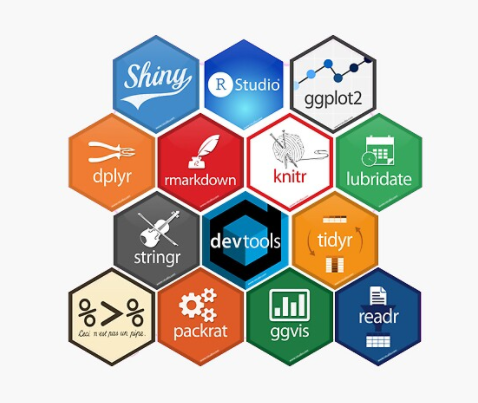# Chapter 1 Preface

This e-book offers generic scripts for conducting core statistical analyses, from calculating statistics to producing tables or graphing results. These scripts should be considered a starting point, not an end point, in your exploration of R.The following chapters cover:

• Getting up and running in R and RStudio
• Using Generic Scripts to Learn R
• Data Prep
• Descriptive Statistics and Data Visualization
• T-Test
• ANOVA
• Cross-Tab
• Correlation and Simple Regression
• Multiple OLS Regression
• Multiple Logistic Regression